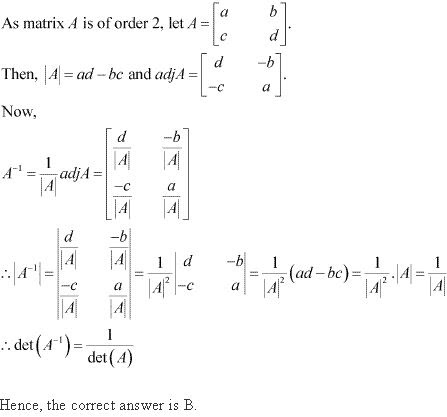# Class 12 Maths NCERT Solutions for Chapter 4 Determinants Exercise 4.5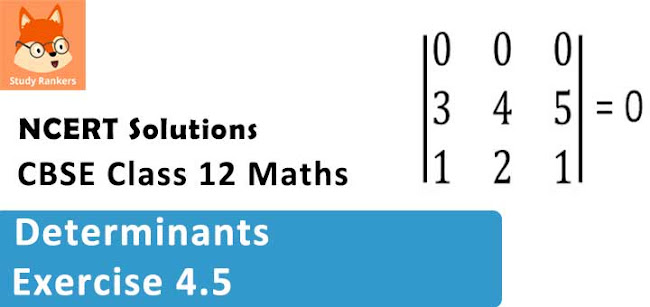### Determinants Exercise 4.5 Solutions

1. Find the adjoint of the matrix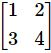Solution

Let A =We have,
A11 = 4, A12 = -3, A21 = -2, A22 = 1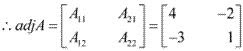2. Find adjoint of each of the matrices.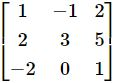Solution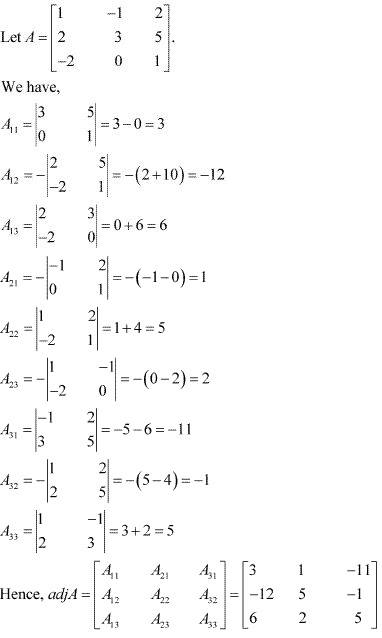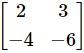Solution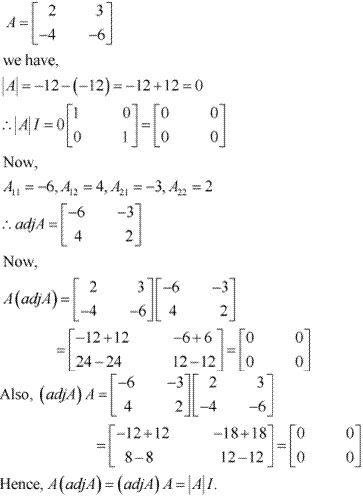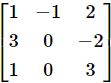Solution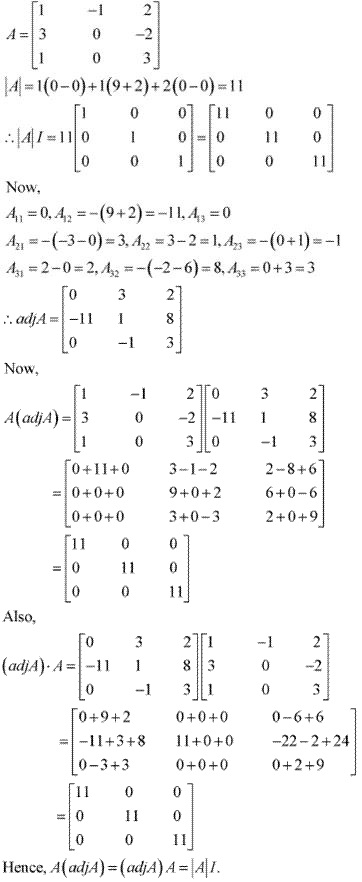5. Find the inverse of each of the matrices (if it exists).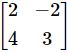Solution
Let A =We have,
|A| = 6 + 8 = 14
Now,
A11 = 3, A12 = -4, A21 = 2, A22 = 2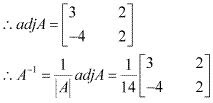6. Find the inverse of each of the matrices (if it exists).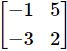Solution
Let A =We have,
|A| = -2 + 15= 13
Now,
A11 = 2, A12 = 3, A21 = -5, A22 = -1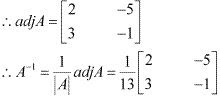7. Find the inverse of each of the matrices (if it exists).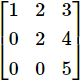Solution
Let A =We have,
|A| = 1(10 - 0) - 2(0 - 0) + 3(0 - 0) = 10
Now,
A11 = 10 - 0 = 10 , A12 = -(0 - 0) = 0, A13 = 0 - 0 = 0,
A21 = -(10 - 0) = -10, A22 = 5 - 0 = 5, A23 = -(0 - 0) = 0
A31 = 8 - 6= 2 , A32 = -(4 - 0) = -4, A33 = 2 - 0 = 2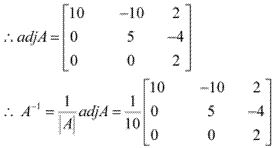8. Find the inverse of each of the matrix (if it exists).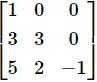Solution
Let A =We have,
|A| = 1(-3 - 0) - 0 + 0 = -3
Now,
A11 = -3 - 0 = -3 , A12 = -(-3 - 0) = 3, A13 = 6 - 15 = -9,
A21 = -(0 - 0) = 0, A22 = -1 - 0 = -1, A23 = -(2 - 0) = -2
A31 = 0 - 0 = 0 , A32 = -(0 - 0) = 0, A33 = 3 - 0 = 3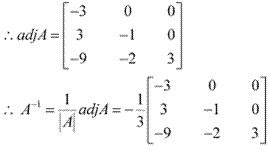9. Find the inverse of each of the matrices (if it exists).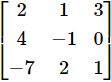Solution
Let A =We have,
|A| = 2(-1 - 0) - 1(4 - 0) + 3(8 - 7)
= 2(-1) - 1(4) + 3(1)
= -2 - 4 + 3
= - 3
Now,
A11 = -1 - 0 = -1 , A12 = -(4 - 0) = -4, A13 = 8 - 7 = 1
A21 = -(1 - 6) = 5, A22 = 2 + 21 = 23, A23 = -(4 + 7) = -11
A31 = 0 - 3 = 3 , A32 = -(0 - 12) = 12, A33 = -2 - 4 = -6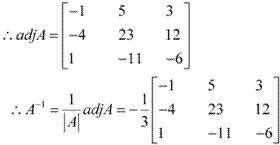10. Find the inverse of each of the matrices (if it exists).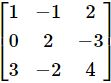Solution
Let A =By expanding along C1 , we have :
|A| = 1(8 - 6) - 0 + 3(3 - 4) = 2 - 3 = -1
Now,
A11 = 8 - 6 = 2 , A12 = -(0 + 9) = -9, A13 = 0 - 6 = -6
A21 = -(-4 + 4) = 0, A22 = 4 - 6 = -2, A23 = -(-2 + 3) = -1
A31 = 3 - 4 = -1 , A32 = -(-3 - 0) = 3, A33 = 2 - 0 = 2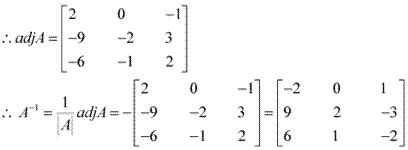11. Find the inverse of each of the matrices (if it exists).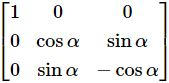Solution
Let A =We have,
|A| = 1(−cos2 α – sin2α) = −(cos2α + sin2 α) = − 1
Now,
A11 = −cos2 α – sin2α = -1  , A12 = 0, A13 = 0
A21 = 0, A22 = −cosα , A23 =  – sinα
A31 = 0 , A32 = – sinα, A33 = cosα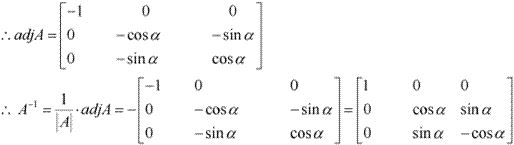12. Let A =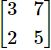and  B =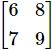. Verify that (AB)-1 = B-1 A-1 .

Solution

Let A =We have,
|A| = 15 - 14 = 1
Now,
A11 = 5, A12 = - 2, A21 = -7, A22 = 3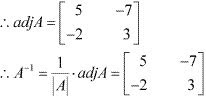Now, Let B =We have,
|B| = 54 - 56 = -2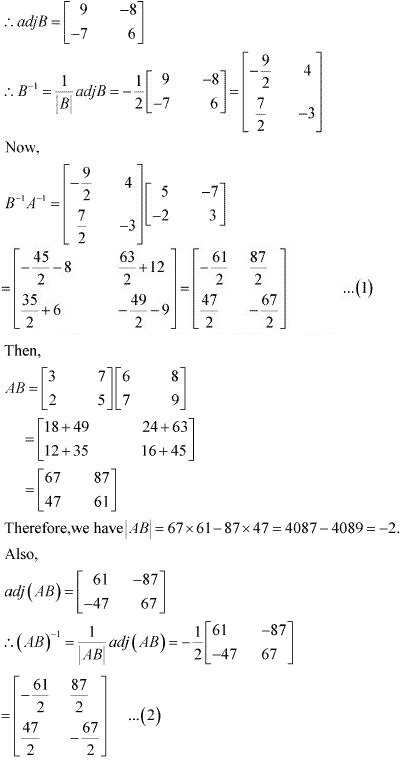From (1) and (2), we have :
(AB)-1 = B-1 A-1
Hence, the given result is proved.

13. If A =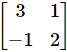show that A2 - 5A + 7I = 0. Hence find A-1.
Solution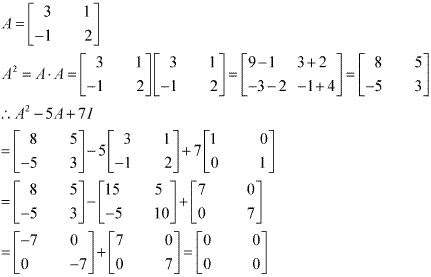Hence, A2 - 5A + 7I = 0.
∴ A . A - 5A = -7I
⇒ A.A(A-1 ) - 5AA-1 = -7IA-1 [Post-multiplying by A-1 as  |A| ≠ 0]
⇒ A(AA-1 ) - 5I = -7A-1
⇒ AI -5I = -7A-1
⇒ A-1 = - (1/7)[A - 5I]
⇒ A-1 = (1/7)[5I - A]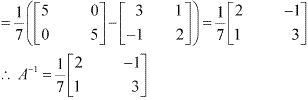14. For the matrix A =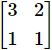find the numbers a and b such that A2  + aA + bI = 0.
Solution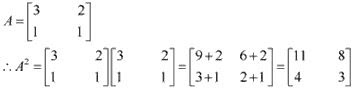Now,
A2 + aA + bI = 0
⇒ (AA)A-1  - aAA-1 = bIA-1  = 0   [Post-multiplying by A-1 as  |A| ≠ 0]
⇒ A(AA-1 ) - aI + b(IA-1 ) = 0
⇒ AI + aI + bA-1 = 0
⇒ A + aI = -bA-1
⇒ A-1 = -(1/b)[A + aI]
Now,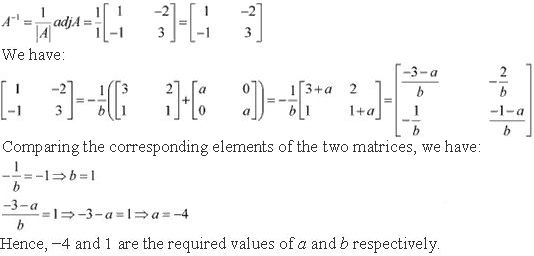15. For the matrix A =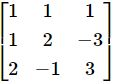show that A3 - 6A2 + 5A + 11I = 0 . Hence, find A-1 .
SolutionA3 - 6A2 + 5A + 11I = 0
⇒ (AAA)A-1  - 6(AA)A-3 + 5AA-1 + 11IA-1  = 0  [Post-multiplying by A-1 as  |A| ≠ 0]
⇒ AA(AA-1 ) - 6A(AA-1 ) + 5(AA-1 ) = 11(IA-1  )
⇒ A2 - 6A + 5I = -11A-1
⇒ A + aI = -bA-1
⇒ A-1 = -(1/11)[A2 - 6A + 5I]  ...(1)
Now,
A2 - 6A + 5I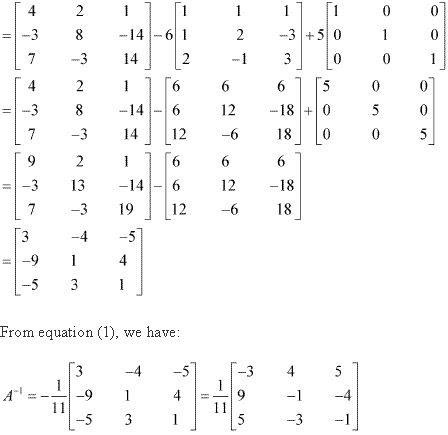16. If A =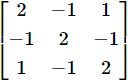verify that A3 - 6A2 + 9A - 4I = 0 and hence find A-1.
Solution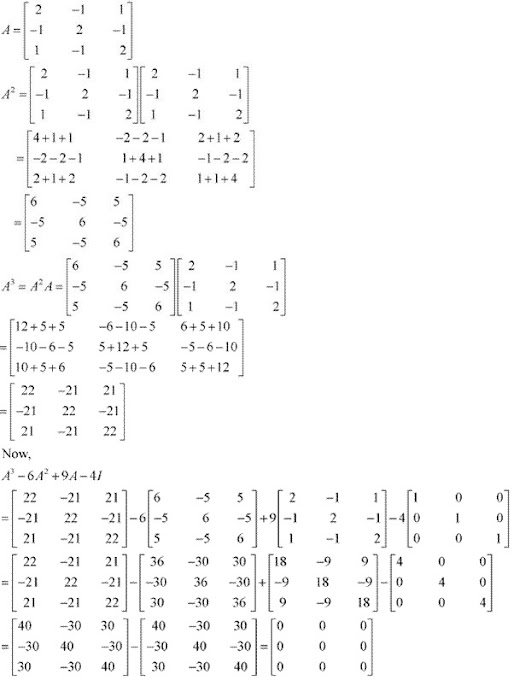∴ A3 - 6A2 + 9A + 4I = 0
Now,
A3 - 6A2 + 9A + 4I = 0
⇒ (AAA)A-1  - 6(AA)A-3 + 9AA-1 + 4IA-1  = 0   [Post-multiplying by A-1 as  |A| ≠ 0]
⇒ AA(AA-1 ) - 6A(AA-1 ) + 9(AA-1 ) = 4(IA-1  )
⇒ AAI - 6AI + 9I = 4A-1
⇒ A2 - 6A + 9I = 4A-1
⇒ A + aI = -bA-1
⇒ A-1 = (1/4)[A2 - 6A + 9I]  ...(1)
A2 - 6A + 9I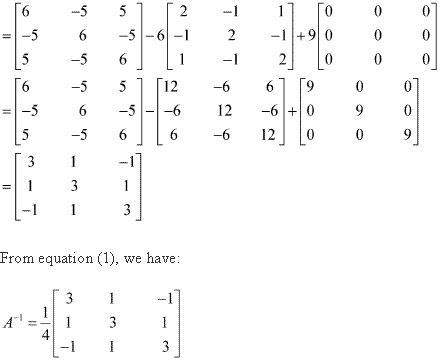17. Let A be a nonsingular square matrix of order 3 × 3. Then |adj A| is equal to
(A) |A |
(B) |A|2
(C) |A|3
(D) 3|A|
Solution
We know that,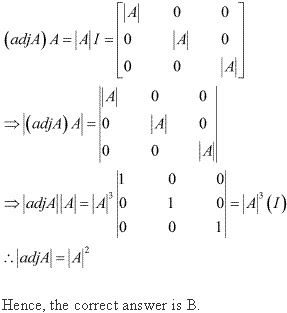18. If A is an invertible matrix of order 2, then det (A−1) is equal to
(A) det (A)
(B) 1/det (A)
(C) 1
(D) 0
Solution
Since A is an invertible matrix, A-1 exists and A-1 = (1/|A|) adj A.